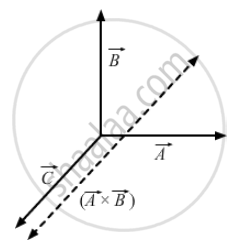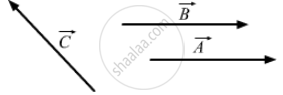Department of Pre-University Education, KarnatakaPUC Karnataka Science Class 11

# If → a , → B , → C Are Mutually Perpendicular, Show that → C × ( → a × → B ) = 0 is the Converse True? - Physics

Sum

If $\vec{A} , \vec{B} , \vec{C}$ are mutually perpendicular, show that  $\vec{C} \times \left( \vec{A} \times \vec{B} \right) = 0$ Is the converse true?

#### Solution

Given: $\vec{A} , \vec{B} \text{ and }\vec{C}$ are mutually perpendicular. $\vec{A} \times \vec{B}$ is a vector with its direction perpendicular to the plane containing $\vec{A} \text{ and } \vec{B}$∴ The angle between $\vec{C} \text{ and } \vec{A} \times \vec{B}$ is either 0° or 180°.
i.e., $\vec{C} \times \left( \vec{A} \times \vec{B} \right) = 0$ However, the converse is not true. For example, if two of the vectors are parallel, then also, $\vec{C} \times \left( \vec{A} \times \vec{B} \right) = 0$So, they need not be mutually perpendicular.

Concept: What is Physics?
Is there an error in this question or solution?

#### APPEARS IN

HC Verma Class 11, Class 12 Concepts of Physics Vol. 1
Chapter 2 Physics and Mathematics
Exercise | Q 16 | Page 29
Share